Example Questions

1 2 3 4 5 7 Next →

A square with width of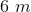is inscribed in a circle. What is the total area inside the circle?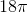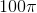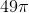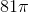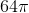Explanation:

We know that each side of the square is 6, so use the Pythagorean Theorem to solve for the diagonal of the square. The diagonal of the square is also the diameter of the circle.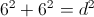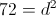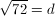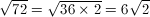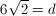Therefore the radius must be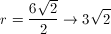Now let's find the area inside the circle using the radius.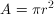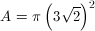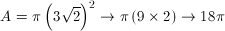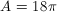meters

Quantity A:

The diagonal of a square with a side-length of.

Quantity B:

The side-length of a square with a diagonal of.

Both quantities are equal.

Quantity B is greater.

The relationship between the two quantities cannot be determined from the information given.

Quantity A is greater.

Both quantities are equal.

Explanation:

Quantity A: The diagonal of a square with a side-length of 7.

Quantity B: The side-length of a square with a diagonal of 14.

Both quantities can be determined, so can the relationship.

Quantity A:

To determine the diagonal of a square, it's important to remember that a diagonal directly bisects a square from corner to corner. In other words, it bisects the corners, creating two triangles with 45:45:90 proportions, with the diagonal serving as the hypotenuses . If you remember your special triangles, then the side-side-hypotenuse measurements have a ratio of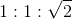In Quantity A, the side-length is 7. Following the proportion: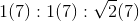The diagonal equals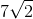.

Quantity B:

We can use the same ratio to figure out quantity B by substituting x for the unknown side-length quantity, which looks like this: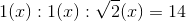To find x in this ratio, just isolate x in the hypotenuse: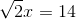Divide by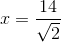Now, how does Quantity A and Quantity B match up?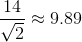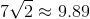On the surface, it looks like the two quanties are equal. But how do we prove it?  Well, we know that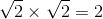.  Therefore, we know that: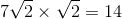Divide both sides by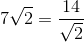Therefore, both quantities are equal.

Quantity A:

The side-length of a square with a perimeter of.

Quantity B:

The side-length of a square with an area of.

The relationship cannot be determined from the information given

Both quantities are equal

Quantity B is greater

Quantity A is greater

Quantity A is greater

Explanation:

The first step to a quantitative comparison is to determine whether it can be solved at all with the given knowledge. Since all you need to find the side-length of a square is the perimeter, the area, OR the diagonal and we have one of each for these two quantities, this relationship can be determined. Thus, "the relationship cannot be determined" is out.

Now, to solve both quantities.  Quantity A can be solved by translating the perimeter into side lengths: the formula for the perimeter of a square is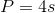, withbeing the side-length, so you just need to divide the perimeter by four.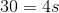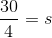Thus, quantity A is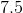.

Quantity B can be solved by translating the area into side lengths: the formula for the area of a square is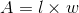, or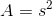, withbeing the side-length, so you just need to find the square root of the area.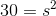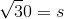Thus, quantity B is roughly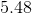.

Therefore quantity A is greater.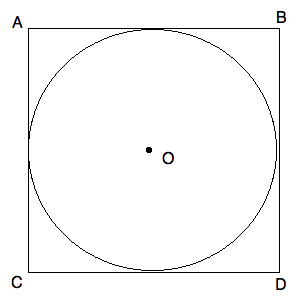Circle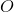has a center in the center of Square.

If the area of Circleis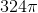, what is the length of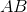?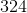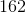Explanation:

If the area of Circleis, we know that the area can be computed using the standard area formula:, usingfor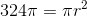Simplifying, we get: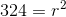We know thatmust be less than. By choosingfor, we find out that this is the radius of our circle. Thus, we know that the diameter of the circle is double this, or. Now, consider the following diagram: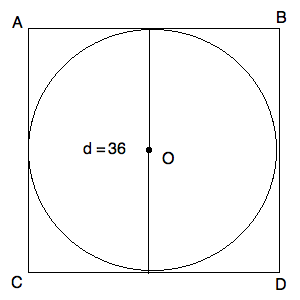Notice that the diameter is the same length as a side of the square. Thus,is equal to.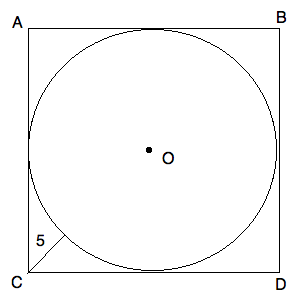Circlehas a center in the center of square.

The line segment marked with lengthlies on the diagonal of the square.

What is the length of side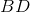?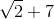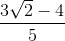This cannot be computed from the given data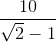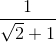Explanation:

You can further fill in your diagram as follows: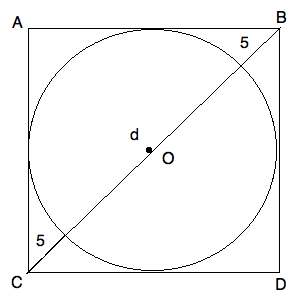Now, we know that the triangle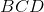is a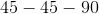triangle. We also know that the length ofand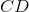must also be equal to the diameter of the circle. (The diameter of the circle will run across the circle horizontally if you draw it that way. This will provide you with a complete side length.) Now, we know that the ratio of the hypotenuse ofto the side must be the same as: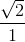For our data, that means: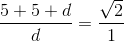Simplifying, we know: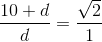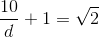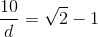Now, make both sides reciprocals: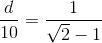Finally, solve: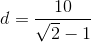Recall, this is both the length of the side and the diameter of the circle. Hence, you have your answer.

1 2 3 4 5 7 Next →

Tired of practice problems?

Try live online GRE prep today.